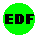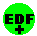European Data FormatSpecs Downloads CompaniesLongintegers and floats

How to store longintegers and floats

EDF and EDF+ are based on 2-byte integer data values. This page specifies how to store longer-integer or floating-point data into these 2-byte integers. The specification is formally part of EDF+.

Reference: Kemp B, Penzel T, Värri AO, Sykacek P, Roberts SJ, Nielsen KD. EDF: a simple format for graphical analysis results from polygraphic SIESTA recordings. J Sleep Research 7, suppl. 2, 1998:132.

Acknowledgement: this transformation was designed in the Biomed-2 project ‘SIESTA’, BMH4-CT97-2040 led by Georg Dorffner (Vienna) and funded by the European Commission, DG XII.

In many cases, the floating point data can be scaled to fit into the available 2byte integer range of -32768 till 32767. The scaling factor can be adapted to the dynamic range of the analysis result even after the analysis was done. Put these scaling factors in the header (digital and physical minimum and maximum) of the analysis file. If such scaling is really impossible because the useful dynamic range of the analysis result is too large, but only then, apply the below logarithmic transformation. The transformation is formally part of EDF+, so EDF+ software will automatically apply the inverse transformation when reading the file. But old EDF software is not aware of this transformation and will show the analysis results on a logarithmic scale.

So really try scaling first! In particular, be aware that no biological signal needs more then 14 bits accuracy, even when sampled by a 22-bit ADC!

The logarithmic transformation
The idea is simple. Integers have constant absolute inaccuracy (the difference between two neighbours), equal to 1. So their relative inaccuracy ranges from 0.03% (at maxint) to 100% (at 1). A logarithmic transformation maps realtype values to 2-byte integer values in such a way that the relative resolution is the same everywhere in a selectable range. Depending on this range, this constant relative resolution is about 0.05% to 1%.

The transformation does not cause any incompatibility with EDF and EDF+. The transformation is logarithmic and symmetric around 0. It has user-selected parameters a and Ymin. It converts floating-point values, Y, to 2-byte integers, N. All float values between -Ymin and Ymin are rounded to N=0. All values of Y larger than Ymin and smaller than –Ymin are logaritmically (with the LN being e-based) converted to N>0 and N<0, respectively. The obtained integers, N, must be exponentially (with the EXP also being e-based) converted back to to the original float value, Y. The relative resolution of this conversion is constant, i.e. Y(N)/Y(N-1) = EXP(a). The exact conversion formulas are:
 Float - to - Integer Integer back to Float If Y>Ymin N=round[LN(Y/Ymin)/a] Y=Ymin*EXP(a*N) If abs(Y)<=Ymin N=0 Y=0 If Y<-Ymin N=-round[LN(-Y/Ymin)/a] Y=-Ymin*EXP(-a*N)
So that EDF and EDF+ software will be aware of the conversion, the ‘physical dimension’ in the EDF or EDF+ fileheader must read ‘Filtered’. The ‘physical maximum’ = ‘digital maximum’ = ‘32767   ‘, and the ‘physical minimum’ = ‘digital minimum’ =’-32767  ‘. Note that digital minimum is -32767, not -32768. The parameters a and Ymin can be chosen freely and have to be specified, together with the dimension of the original signal, in the ‘prefiltering’ field of the header. This field should contain ‘sign*LN[sign*(physical dimension)/(Ymin)]/(a)’ in which ‘physical dimension’ and the values of a and Ymin are coded in arrays of  8 ASCII characters (left-justified and filled out with spaces). For example if a=0.002 and Ymin=0.01 and the physical dimension of Y was ‘uV      ‘, then the prefiltering field should contain: sign*LN[sign*(uV      )/(0.01    )]/(0.002   ).

The accuracy of the transformation
Y(1) and Y(-1) are the smallest float values that can be distinguished from 0. So, if Ymin=0.001, then all values between -0.001 and +0.001 are rounded to 0.
Float values outside this range are represented with the selected accuracy, a. The relative accuracy is Y(N)/Y(N-1) = EXP(a). For instance if a=0.005 (that is 0.5%) then floating point values that differ less than 0.5% from each other are rounded to the same value. So, values 1.1226 - 1.1275 are rounded to 1.125, values 1.1276 - 1.1325 are rounded to 1.130 and so on. Also, values 0.009824 - 0.009875 are rounded to 0.0985 and values 9824 - 9875 are rounded to 9850.

Examples
 a Ymin Accuracy of Y Range of Y (+ and -) 0.005 0.001 0.5 % 0.001 till 1.42E+68 0.005 0.00001 0.5 % 0.00001 till 1.42 E+66 0.0005 0.1 0.05 % 0.1 till 1.30E+6 0.0005 1 0.05 % 1 till 1.30E+5

The top rows show that floating-point variables can be stored in EDF+ with an accuracy of 0.5%. Try other values of a and Ymin in this spreadsheet.